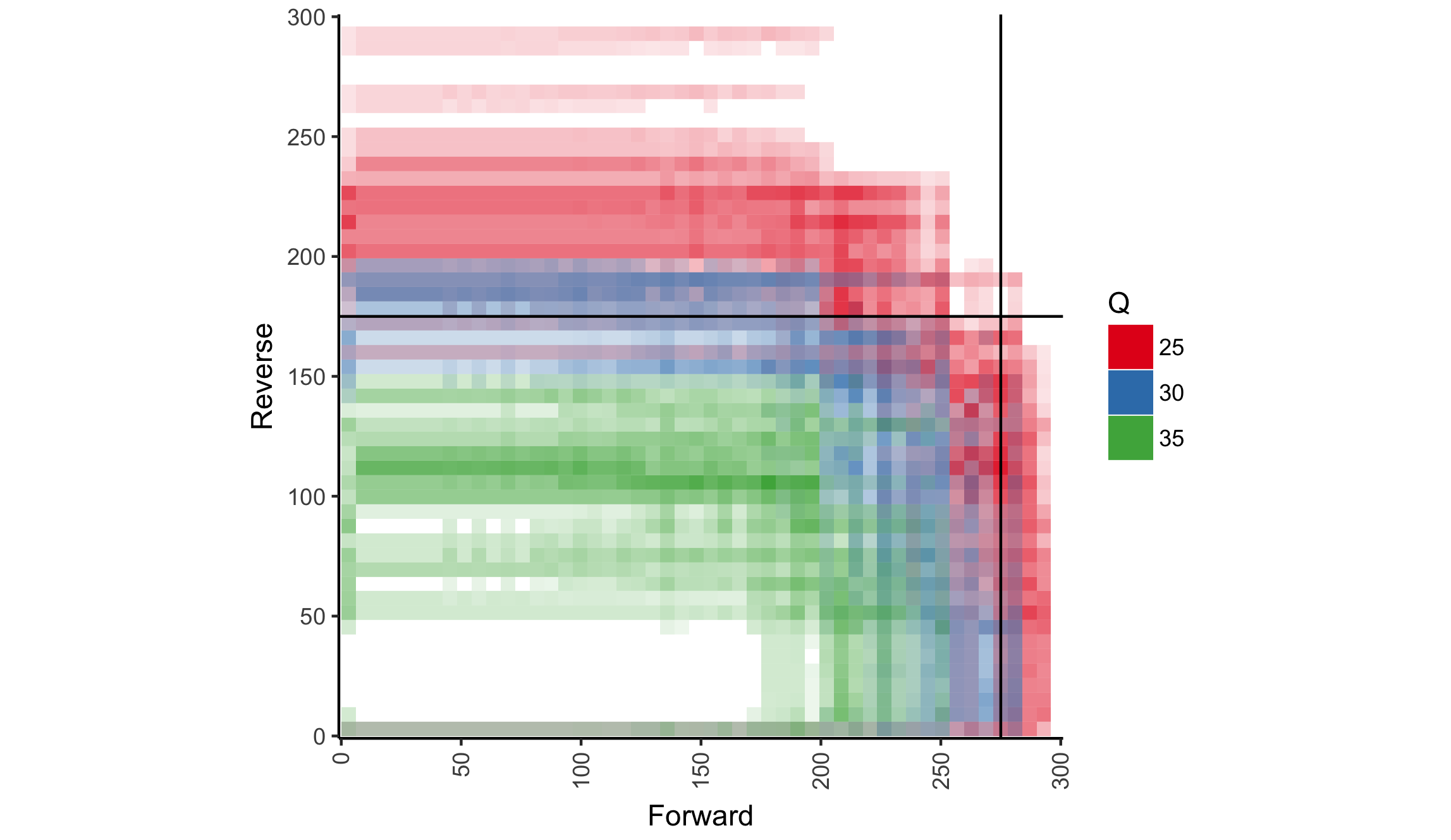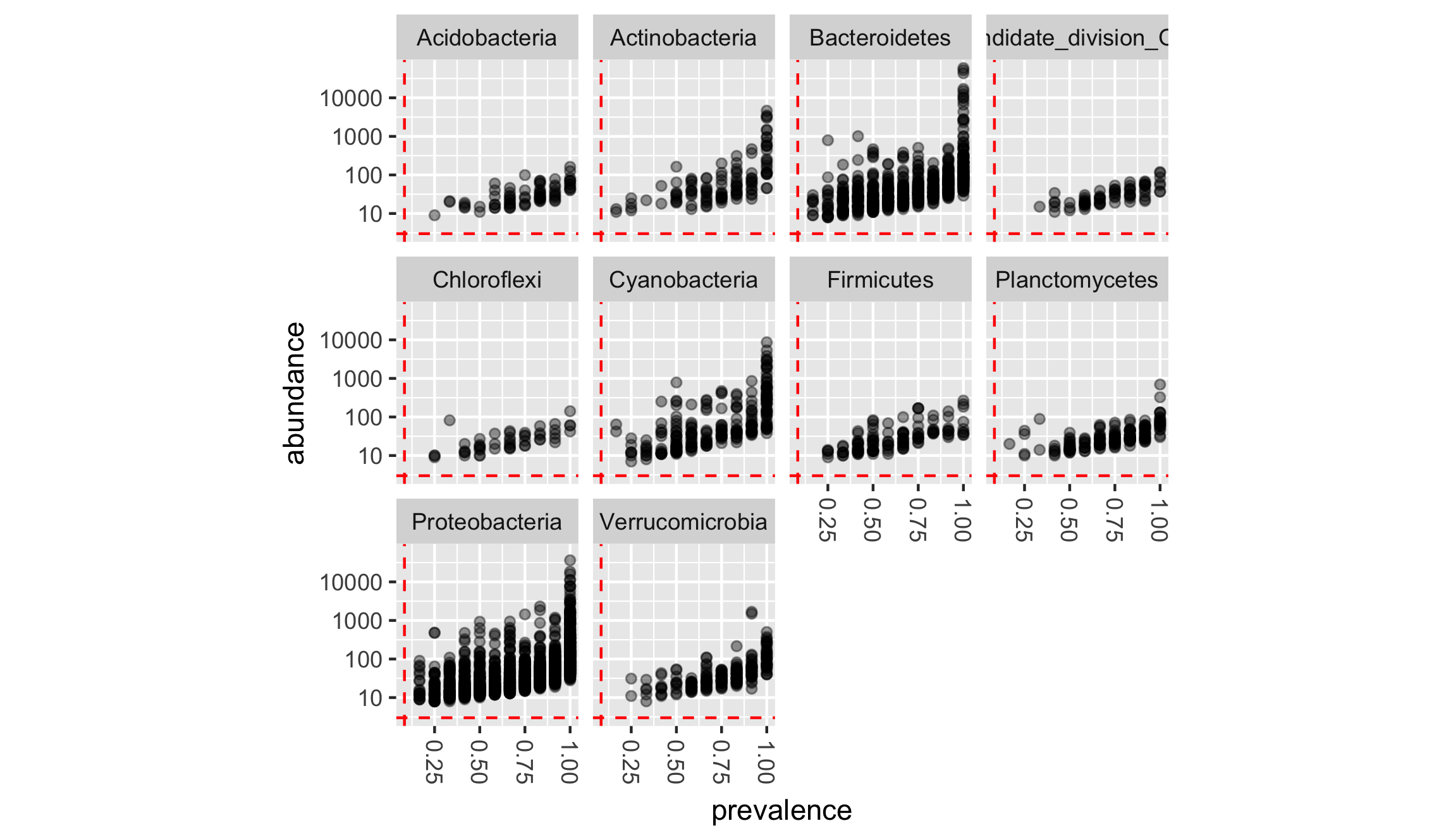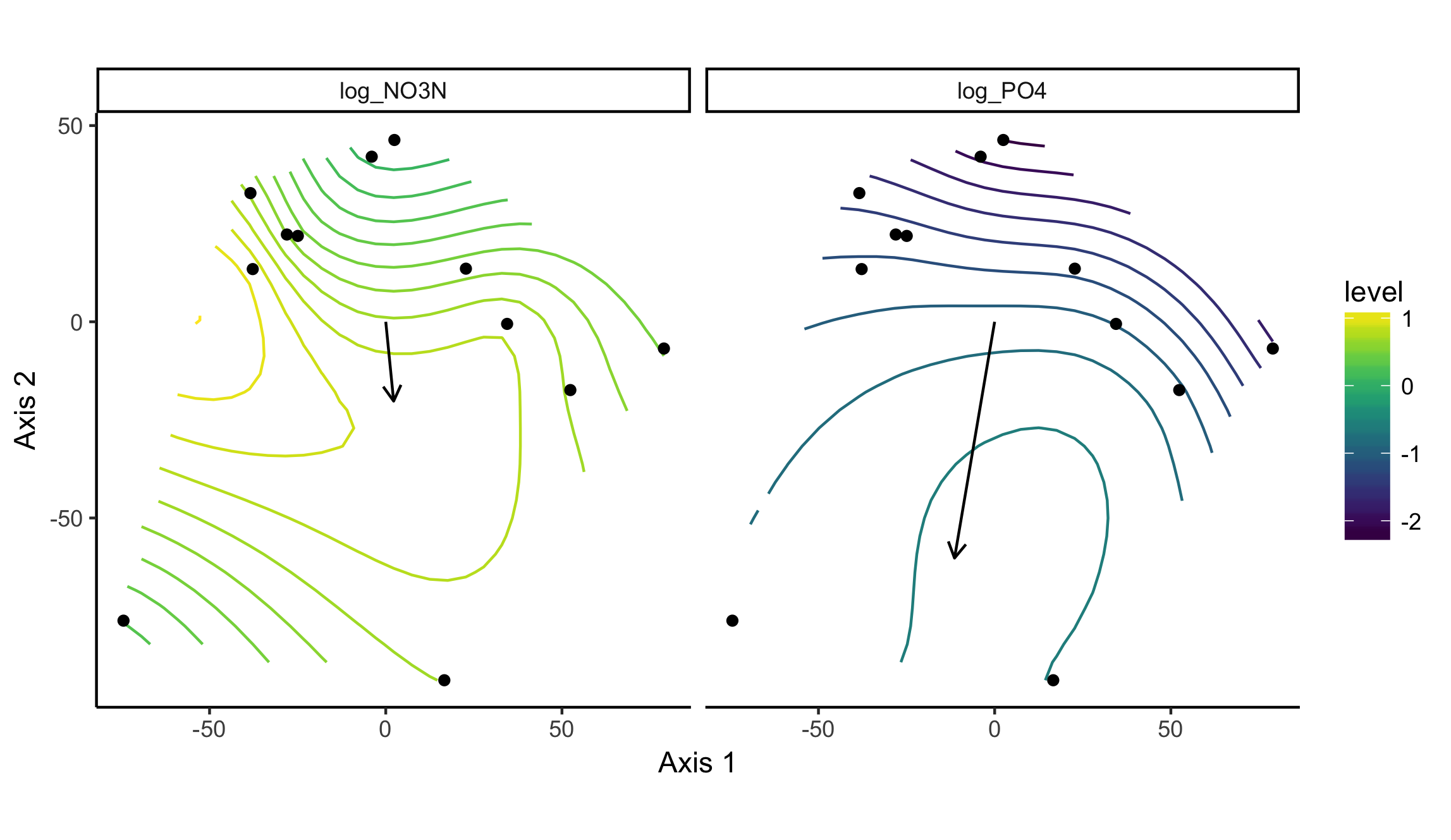# Using the theseus R package

## Introduction

We will be using the dataset WWTP_Impact from Price et. al. (2017) to illustrate the basic functionality within the theseus package. To get started, let’s load theseus, phyloseq, and ggplot2 and get the WWTP_Impact dataset.

``````library(theseus)
library(phyloseq)
library(ggplot2)

data('WWTP_Impact')``````

WWTP_Impact is a phyloseq object containing 16S rDNA amplicon sequencing data from samples collected at 6 sites on 2 days (12 total samples) along Wissahickon Creek and Sandy Run in southeastern Pennsylvania, USA. Sequencing data was processed as described in Price et. al. (2016), and combined with chemical data to create a phyloseq object. The raw data and scripts used to generate this phyloseq object (as well as the phyloseq object itself) can be obtained from the author’s GitHub repository.

## qualcontour - generate a contour plot of quality scores of raw reads.

Trimming and quality filtering is one of the first steps taken when analyzing amplicon sequencing results. Indiscriminate trimming can cause a host of issues for downstream processes. Function qualcontour is intended to assist the user with deciding where trimming should be performed.

qualcontour’s (quality contour) two required arguments are character vectors of the file paths for forward (f_path) and reverse (r_path) reads. qualcontour tabulates the distribution of quality scores at each read cycle for the forward and reverse reads independently and then averages (arithmetic mean) the quality scores for each (forward/reverse) cycle combination. These values are then plotted as a ggplot2 object.

``````fns <- sort(list.files(file.path(system.file(package='theseus'), 'testdata'), full.names=TRUE))
f_path <- fns[grepl('R1.fastq.gz', fns)]
r_path <- fns[grepl('R2.fastq.gz', fns)]
p.qc <- qualcontour(f_path, r_path, n_samples=2, verbose=TRUE, percentile=.25, nc=1)

p.qc + geom_hline(yintercept=175) + geom_vline(xintercept=275)``````Using this visualization, we can see that reverse read quality drops earlier, and more gradually when compared with the forward read quality. Selecting the appropriate trimming location can be tricky and there are multiple ways to approach the challenge. Two places (or rules) to start with are:
* trimming where a severe/sudden drop in read quality occurs, such as at ~275 for the forward read
* trimming where the quality scores drop below a certain (sometimes arbitrarily selected) value, such as ~175 in the reverse reads, where we estimate that read quality hit Q=30.

## prev - prevalence filtering

Low count taxa are often filtered from OTU tables to reduce possible error or noise. Examination of the raw (unfiltered) OTU table should be carried out to ensure that appropriate thresholds for prevalence (number of samples a taxa was observed in) and abundance (the total number of times a taxa was observed) are being selected. Function prev (prevalance) plots each taxa according to thier prevalance and abundance within the dataset.

``````p.prev <- prev(WWTP_Impact, taxon="Phylum", n_taxa=10)
p.prev``````## pstoveg_otu and pstoveg_sd: converting objects between phyloseq and vegan

vegan provides some functionality that phyloseq does not, for example statistical tests for ordination objects. The functions pstoveg_otu and pstoveg_sd are short and simple helper functions to help the user move or convert phyloseq objects to vegan-compatible objects.

vegan wants OTU tables to have taxa as columns and samples/sites as rows, while phyloseq can handle either orientation. *psotuveg_otu** will test to see if taxa are rows and carry out the transposition if required.

``````dim(otu_table(WWTP_Impact))
taxa_are_rows(WWTP_Impact)
otu <- pstoveg_otu(WWTP_Impact)
dim(otu)

data(GlobalPatterns, package='phyloseq')
dim(otu_table(GlobalPatterns))
taxa_are_rows(GlobalPatterns)
otu.gp <-pstoveg_otu(GlobalPatterns)
dim(otu.gp)``````

Extracting sample_data() from a phyloseq object is just as easy.

``````dim(sample_data(WWTP_Impact))
sampdat <- pstoveg_sd(WWTP_Impact)
dim(sampdat)

data(GlobalPatterns, package='phyloseq')
dim(sample_data(GlobalPatterns))
sampdat.gp <-pstoveg_sd(GlobalPatterns)
dim(sampdat.gp)``````

If vegan is used to carry out OTU transformations, users can easily pull the transformed data back into the phyloseq object.

``````wwtp <- WWTP_Impact
otu.ra <- vegan::decostand(otu, method='total')
otu_table(wwtp) <- otu_table(otu.ra, taxa_are_rows = taxa_are_rows(WWTP_Impact))``````

The same can be said for any altered or added values of the sample data.

``````sampdat.altered <- sampdat
sampdat.altered\$TotDisP_PercentMax <- vegan::decostand(sampdat\$TotDisP, method='max')
sample_data(wwtp) <- as.data.frame(sampdat.altered)``````

## envtoverlay - Fitting environmental vectors and surfaces into ordination space

The calculations for unconstrained ordination does not take environmental or sample metadata into account. The vegan functions envfit and ordisurf attempt to fit environmental descriptors into ordination space and display them as vectors and surfaces respectively. Gavin Simpson, one of vegan’s authors, has a great blog explaining how these functions work and are interpreted. Jari Oksanen has provided several vignettes on vegan’s homepage that also discuss the application of these functions.

Function envtoverlay (environmental variable overlay) takes advantage of ggplot2 to make these plots (usually generated in base::plot) a little prettier and user friendly, particularly when the user would like to present the results as a faceted plot.

``````cv <- c('log_NO3N', 'log_PO4')
envtoverlay(WWTP_Impact, covariates=cv)``````## constord

Function constord (constrained ordination) carries out constrained ordination on a phyloseq object and plots the results. Constrained correspondence analysis (‘CCA’) and redundancy analysis (‘RDA’) are the two methods currently implemented within this function.

``constord(PS=WWTP_Impact, formula=~ log_NO3N + log_PO4, method='RDA', facets=Position~Location, scaling=2)``